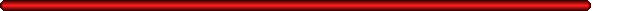SB 0002 Interest calculated by actuarial method on prepayment of loansSponsor: Schneider LR Number: S0443.01I Fiscal Note: 0443-01 Committee: Financial and Governmental Organization Last Action: 01/25/99 - Hearing Conducted S Financial & Governmental Journal page: Organization Committee Title: Effective Date: August 28, 1999Full Bill Text | All Actions | Available Summaries | Senate Home Page | List of 1999 Senate BillsCurrent Bill Summary

SB 2 - This act provides that upon the prepayment of any retail installment contract, premium finance contract, credit life, accident or sickness insurance policy, small loan or retail credit sale, a refund of prepaid interest must be calculated by the actuarial method. The "sum of the digits" or "rule of 78" method of calculating prepaid interest refunds is prohibited.

When using the sum of the digits method to calculate a refund of prepared interest, the denominator is always the sum of the total number of months in the loan added together in ascending order. For example, in a one-year loan the denominator is always 78 (1+2+3+4+....+12) and in a two-year loan the denominator is always 300 (1+2+3+4+....+24). A simpler way to calculate the denominator is to multiply the number of months in the loan by the number of months in the loan plus 1 and divide the result by 2. For example, in a one-year loan the denominator is always 78 (12x13/2), in a two year-loan the denominator is always 300 (24x25/2), and in a three-year loan the denominator is always 666 (36x37/2).

The numerator in the sum of the digits method is always the sum of the total number of months in the loan that have expired before payoff added together in descending order. For example, in a one-year loan with three months expired the numerator is always 33 (12+11+10), and with four months expired the numerator is always 42 (12+11+10+9). In a two-year loan with three months expired the numerator is always 69 (24+23+22) and with four months expired the numerator is always 90 (24+23+22+21).

The resulting fraction is applied to the finance charge to calculate the refund.

The "sum of the digits" or "rule of 78" method of calculating prepaid interest refunds front-end loads interest and therefore favors the lender because the borrower pays more interest in the early months of a financial contract than would have been paid using the actuarial method.

In contrast, the actuarial method of calculating prepaid interest refunds approximates the interest actually earned on a day-by-day basis. For a one year loan the interest is 1/365 per day. The actuarial method treats both the lender and the borrower fairly.
RUSS HEMBREE